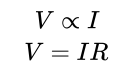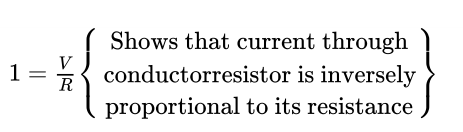# Ohm’s law

George Simon Ohm found the relationship between the current (I) flowing through a conductor and potential difference (V) across the terminals of a conductor using the circuit diagram. He observed that the potential difference and current varied linearly.

Ohm’s Law stated that the electric current flowing through a conductor is directly proportional to the potential difference across its ends, under standard temperature and pressure conditions.Where “R” is the proportionality constant for the given metal at given temperature and is called resistance. The graph between V and I is always straight line with slope equal to R.

# Resistance

It is the property of a conductor that opposes the flow of current. It is represented by ‘R’ and symbol is Ω.

SI unit of resistance is “Ohm”.

# 1 Ohm

The resistance of a conductor is said to be one Ohm when the potential difference across the conductor is 1V and the current flowing through it is 1A.

V = IRSo to increase or decrease the current accordingly in the circuit a component used is called “Rheostat”, that regulates the current without changing potential difference. Represented by “Rh”. If a conductor has less Resistance, then more current will flow through it.

## Factors on Which Resistance of a Conductor Depends

• On its length (L): (Resistance) R Ω L (Directly prop. to length)
• On its cross-sectional area (A)
• On the nature of the material.
• For a material irrespective of length and area, the resistivity is a constant.

## The resistivity of a Material varies with Temperature

Resistivity is the measure of the resistance of a material. In other words, up to what extent will the material resist current flow.

The resistivity of an alloy (a homogeneous mixture of metals) is generally higher than of its constituent metals. Example: Constantan (the alloy of Cu & Ni)

Alloys have high resistivity and do not oxidize (burn) readily at high temperature, for this reason, they are commonly used in electrical heating devices, like electric iron, heater, toasters etc. For example, “Tungsten” as the filament of an electric bulb.

Click on a star to rate it!

Average rating / 5. Vote count:

No votes so far! Be the first to rate this post.